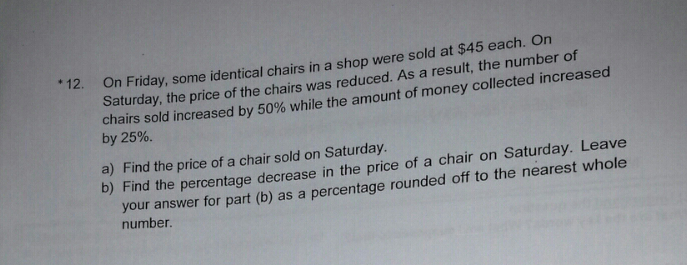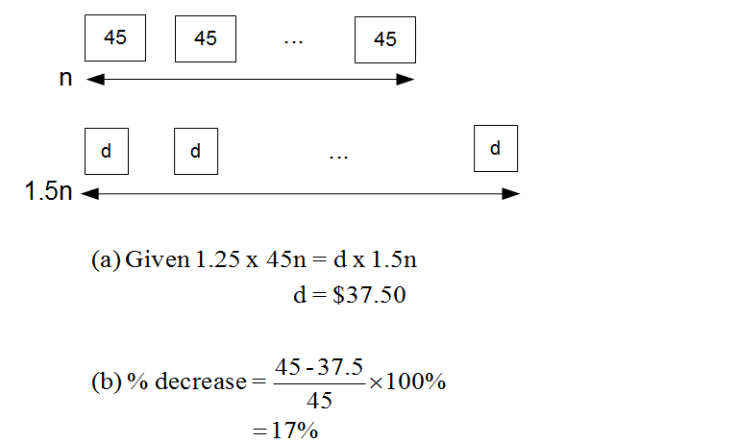QuestionNormal During Sale units sold Let 2u –> 50% more = 3u unit price 45 Takings 90u –> 25% more = 112.5u

(a) Sale price = 112.5 / 3 = \$37.50

(b) % decrease = (decrease / original ) x 100%

= (45-37.5)/45 x 100%

=16.67% (suggest to keep to 2 decimals in working, especially if it is necessary. See *Note)

= 17% (nearest whole number in percentage)

*Note: For example, if anwer is 28.46%.

A student could be writing…………. = 28.5% = 29% (nearest whole number) -wrong.

which correct answer should be …….= 28.46% = 28% (nearest whole number) – correct.